# Atomic TheoryPage 13

#### WATCH ALL SLIDES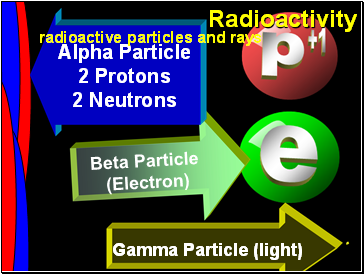Gamma Particle (light)

Beta Particle

(Electron)

Alpha Particle

2 Protons

2 Neutrons

Slide 177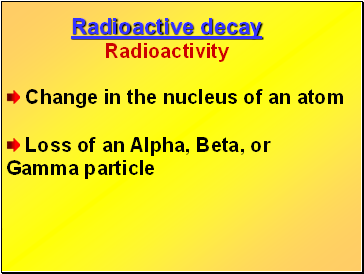Change in the nucleus of an atom

Loss of an Alpha, Beta, or Gamma particle

Slide 178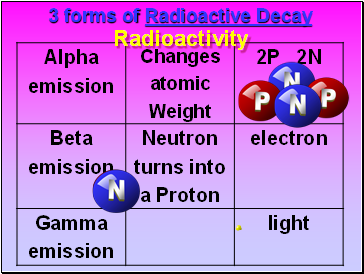Slide 179the Mole !

It’s time to learn about . . .

Slide 180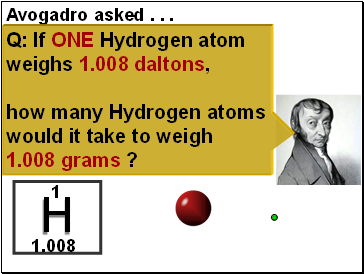H

1.008

1

Q: If ONE Hydrogen atom weighs 1.008 daltons,

how many Hydrogen atoms would it take to weigh 1.008 grams ?

Slide 181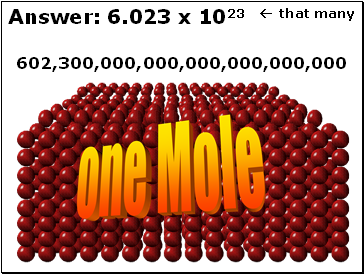Answer: 6.023 x 1023  that many

602,300,000,000,000,000,000,000

one Mole

Slide 182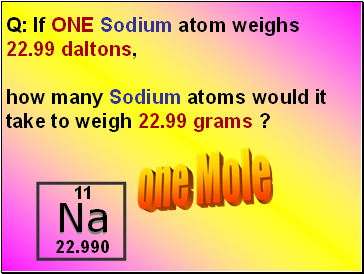Na

22.990

11

Q: If ONE Sodium atom weighs

22.99 daltons,

how many Sodium atoms would it take to weigh 22.99 grams ?

one Mole

Slide 183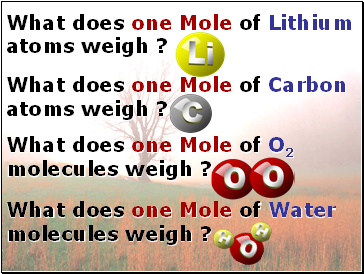What does one Mole of Lithium atoms weigh ?

What does one Mole of Carbon atoms weigh ?

What does one Mole of Water molecules weigh ?

What does one Mole of O2 molecules weigh ?

Slide 184Six munths ago

I cudnt evun spelt chemissed.

An now I are one.

Slide 185